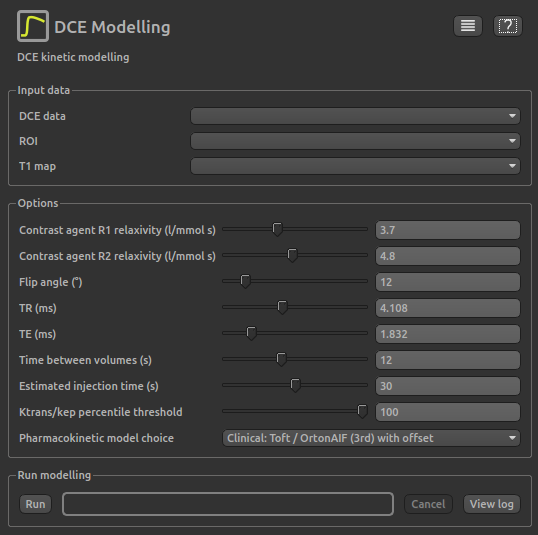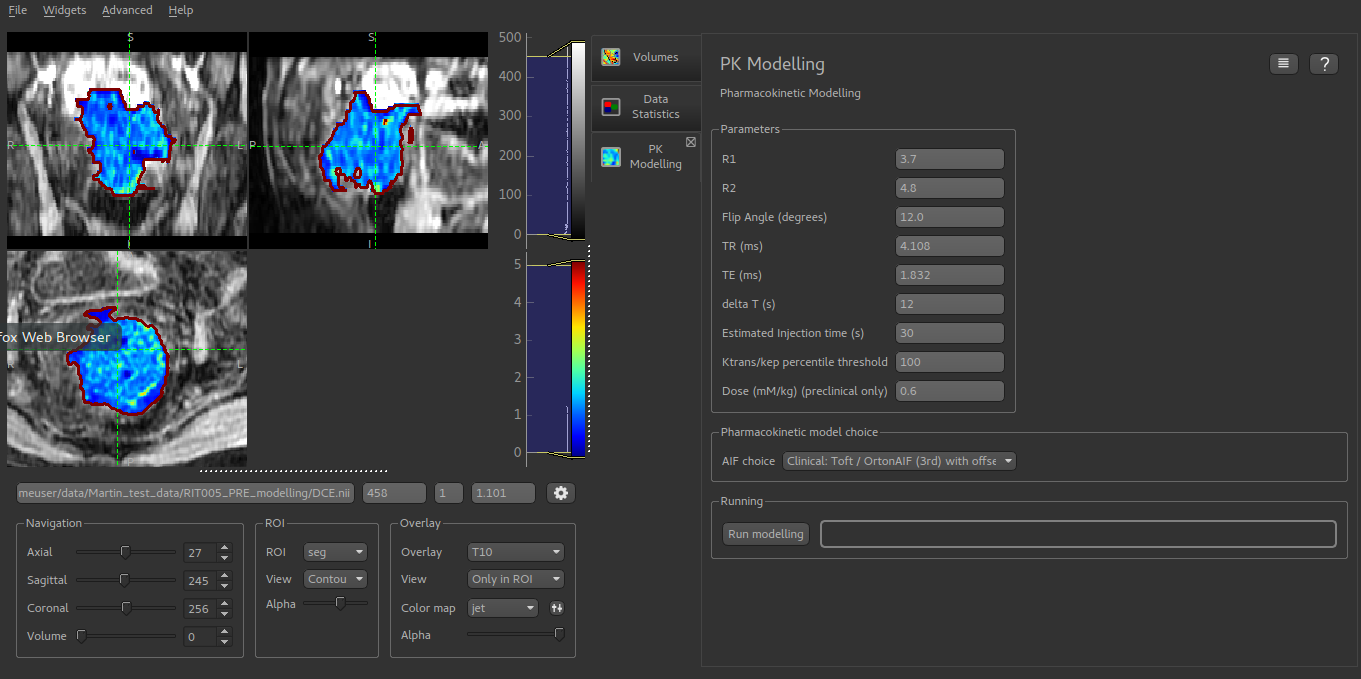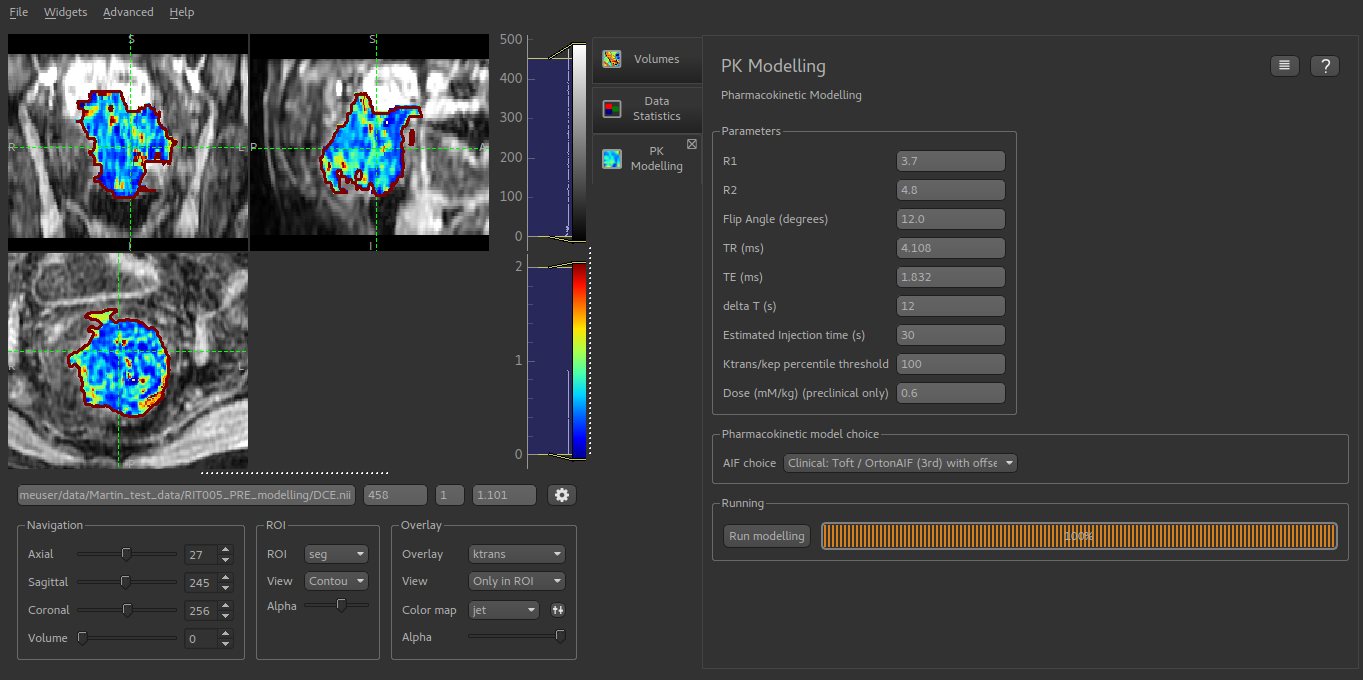# Least-squares DCE modelling¶

The DCE modelling widget performs pharmacokinetic modelling for Dynamic Contrast-Enhanced MRI (DCE) using the Tofts model. Fitting is performed using a simple least-squares technique which limits the range of parameters which can be inferred and the complexity of models which can be implemented. For a more flexible DCE modelling process see Bayesian DCE modelling.## Input data¶

• DCE data is used to select the data set containing the 4D DCE time series
• ROI is used to select the region of interest data set
• T1 is used to select a T1 map which is required for the modelling process. This might be derived from VFA images using the T1 widget or by some other method, e.g. saturation recovery.

## Options¶

• Contrast agent R1/R2 relaxivity should be the T1 and T2 relaxivites from the manufaturer’s documentation. A list of commonly used agents and their relaxivities is also given at List of relaxivities.

• Flip angle is defined by the acquisition parameters and should be given in degrees.

• TR is the repetition time for the acquisition and should be given in milliseconds.

• Similarly TE is the echo time for the acquisition and should be given in milliseconds.

• Time between volumes should be given in seconds. In some cases this may not be fixed as part of the acquisition protocol, but instead a series of volumes acquired, each with the time at which it was acquired. In this case you must determine a sensible time difference to use, for example by dividing the total acquisition time by the number of volumes acquired.

• Estimated injection time is the time delay between the first acquisition and the introduction of the DCE contrast agent in seconds. The latter is often not given immediately in order to establish a baseline signal.

• ktrans / kep percentile threshold limits the maximum value of $$K_{trans} / K_{ep}$$. This is equal to V_e and hence in theory should never exceed 1.0. By reducing this value the effective maximum value of V_e can be limited.

• Pharmacokinetic model choice selects the combination of model an AIF to use in the modelling. Choices available are:

• Clinical Tofts/Orton - Tofts model using Orton (2008) AIF.
• Clinical Tofts/Orton - As above but with baseline signal offset (recommended)
• Preclinical Tofts/Heilman - Tofts model with preclinical AIF from Heilman
• Preclinical Ext Tofts/Heilman Extended Tofts model with preclinical AIF from Heilman

### Screenshots¶

Start of modelling, showing loaded T10 mapModelling complete with newly generated :math:K_{trans} map